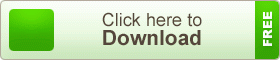# JSC Science Note 7th Chapter Earth and Gravity

JSC Science Note 7th Chapter Earth and Gravity. This means that the gravity of Earth at the equator is 9.789 m/s2, while the force of gravity at the poles is 9.832 m/s2. In other words, you weigh more at the poles than you do at the equator because of this centripetal force, but only slightly more. The gravity of Earth, which is denoted by g, refers to the acceleration that is imparted to objects due to the distribution of mass within the Earth.

In SI units this acceleration is measured in meters per second squared (in symbols, m/s2 or m·s−2) or equivalently in newtons per kilogram (N/kg or N·kg−1). Near the Earth’s surface, gravitational acceleration is approximately 9.8 m/s2, which means that ignoring the effects of air resistance, the speed of an object falling freely will increase by about 9.8 meters per second every second. This quantity is sometimes referred to informally as little g (in contrast, the gravitational constant G is referred to as big G)

## JSC Science Note 7th Chapter Earth and Gravity###The precise strength of Earth’s gravity varies depending on location. The nominal “average” value at the Earth’s surface, known as standard gravity is, by definition, 9.80665 m/s2. This quantity is denoted variously as gn, ge (though this sometimes means the normal equatorial value on Earth, 9.78033 m/s2), g0, gee, or simply g (which is also used for the variable local value). The weight of an object on the Earth’s surface is the downwards force on that object, given by Newton’s second law of motion, or F = ma (force = mass × acceleration). Gravitational acceleration contributes to the total acceleration, but other factors, such as the rotation of the Earth, also contribute, and, therefore, affect the weight of the object.

teachingbd24.com is such a website where you would get all kinds of necessary information regarding educational notes, suggestions and questions’ patterns of school, college, and madrasahs. Particularly you will get here special notes of physics that will be immensely useful to both students and teachers. The builder of the website is Mr. Md. Shah Jamal Who has been serving for 30 years as an Asst. Professor of BAF Shaheen College Dhaka. He expects that this website will meet up all the needs of Bengali version learners /students. He has requested both concerned students and teachers to spread this website home and abroad.

বিজ্ঞান বিষয়ের অন্যান্য অধ্যায়ের নোটের জন্য এখানে ক্লিক করুন।